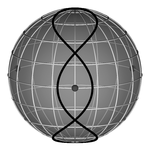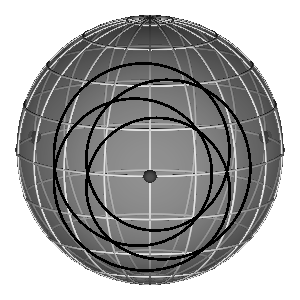TOPOLOGICAL CLASS

DATA AND IMAGES PREPARED BY MARIJA JANKOVIĆ

CLICK ON THE ICON FOR ORBITS...ROGER BROUCKEMICHEL HENONSatellites - MARIJA JANKOVIĆ

## Commentary

- All B-H-H solutions belong to the same topology class: a

- All of these solutions' initial conditions exhibit same symmetry so that their initial conditions are of the form: coordinates $$(x_1,0),(x_2,0),(x_3,0)$$, velocities $$(0,\dot{y}_1),(0,\dot{y}_2),(0,\dot{y}_3)$$

- Initial conditions for the satellites are parametrized using Jacobi coordinates and total angular momentum L: $$a=(x_1-x_2)/\sqrt{2}$$, $$c=(v_{y1}-v_{y2})/\sqrt{2}$$, $$b=(x_1+x_2-2x_3)/\sqrt{6}=1$$, $$d=(v_{y1}+v_{y2}-2v_{y3})/\sqrt{6}=L-ac$$.

- Body masses in Broucke's and Henon's original papers equal $$1/3$$, while all i.c. data given here have been rescaled so that mass equals 1 while the period remains the same

- Henon solutions are relatively periodic, i.e. periodic up to a finite rotation through a certain angle. Their motion in real space is shown in the corotating coordinate system in which all orbits are closed (see  for details)

- Broucke A solutions and all of the Henon solutions are different from Broucke R solutions by the direction of the two rotations (A - retrograde, R - direct)

- Broucke solutions: R8, R9, R10, R13, A15, A8, A9, A10, A14, A15 seem to be "semi-choreographies" where two bodies move on the same trajectory and the third one goes its own way

<<< BACK TO THE GALLERY